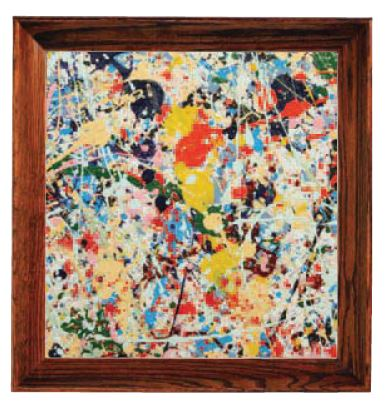Homework Explained - Math Practice 101Dear guest, you are not a registered member. As a guest, you only have read-only access to our books, tests and other practice materials.

As a registered member you can:

Registration is free and doesn't require any type of payment information. Click here to Register.
Go to page:
Chapter 1: Real Numbers; Lesson 1:Rational and Irrational Numbers

### 1.1 Independent Practice

• Question 20

A $$\frac{7}{16}$$-inch-long bolt is used in a machine. What is the length of the bolt written as a decimal?

•  -inch-long
• Question 21

The weight of an object on the moon is $$\frac{1}{6}$$ its weight on Earth. Write $$\frac{1}{6}$$ as a decimal.

• Question 22

The distance to the nearest gas station is $$2\frac{4}{5}$$ kilometers. What is this distance written as a decimal?

• Question 23

A baseball pitcher has pitched $$98\frac{2}{3}$$ innings. What is the number of innings written as a decimal?

• Question 24

A heartbeat takes 0.8 second. How many seconds is this written as a fraction?

• Question 25

There are 26.2 miles in a marathon. Write the number of miles using a fraction.

• Question 26

The average score on a biology test was $$72.\overline{1}$$ . Write the average score using a fraction.

• Question 27

The metal in a penny is worth about 0.505 cent. How many cents is this written as a fraction?

• Question 28

Multistep An artist wants to frame a square painting with an area of 400 square inches. She wants to know the length of the wood trim that is needed to go around the painting.a. If x is the length of one side of the painting, what equation can you set up to find the length of a side?

•  $$x^2$$=
• Question 28

b. Solve the equation you wrote in part a. How many solutions does the equation have?

•  x=$$\pm$$
• Question 28

c.  Do all of the solutions that you found in part b make sense in the context of the problem? Explain.

• Type below:
• Question 28

d. What is the length of the wood trim needed to go around the painting?

•  P= inches

Yes, email page to my online tutor. (if you didn't add a tutor yet, you can add one here)